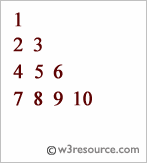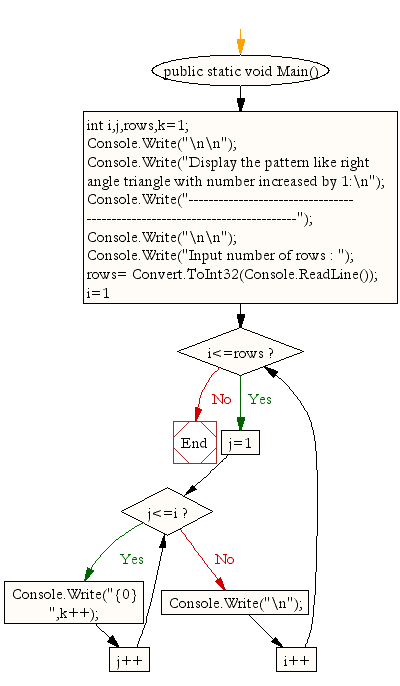﻿ C# - Pattern as a right angle triangle with the number# C# Sharp Exercises: Display the pattern like right angle triangle with number increased by 1

## C# Sharp For Loop: Exercise-12 with Solution

Write a program in C# Sharp to make such a pattern like right angle triangle with number increased by 1. The pattern is as follows :

```1
2 3
4 5 6
7 8 9 10
```Sample Solution:-

C# Sharp Code:

``````using System;
public class Exercise12
{
public static void Main()
{
int i,j,rows,k=1;

Console.Write("\n\n");
Console.Write("Display the pattern like right angle triangle with number increased by 1:\n");
Console.Write("---------------------------------------------------------------------------");
Console.Write("\n\n");

Console.Write("Input number of rows : ");
for(i=1;i<=rows;i++)
{
for(j=1;j<=i;j++)
Console.Write("{0} ",k++);
Console.Write("\n");
}
}
}
```
```

Sample Output:

```Display the pattern like right angle triangle with number increased by 1:
---------------------------------------------------------------------------
Input number of rows : 5
1
2 3
4 5 6
7 8 9 10
11 12 13 14 15
```

Flowchart:C# Sharp Code Editor:

Contribute your code and comments through Disqus.

What is the difficulty level of this exercise?

Test your Programming skills with w3resource's quiz.

﻿